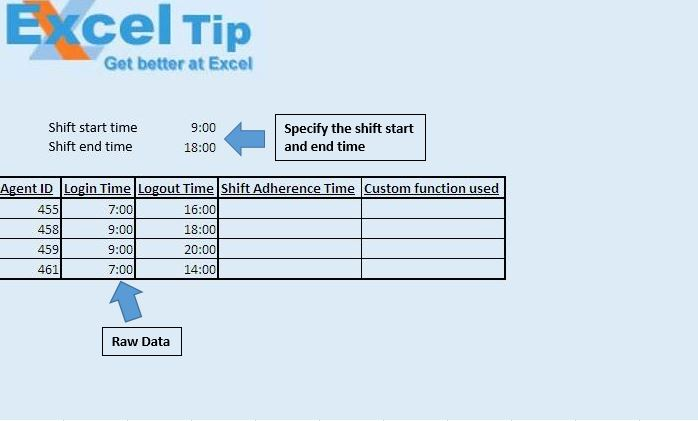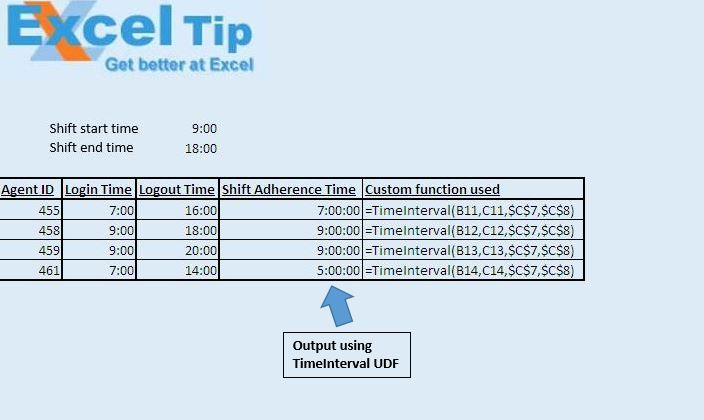# Calculating shift adherence time for given shift using VBA in Microsoft Excel

In this article, we will create a custom function or UDF to calculate the shift adherence time for the agents.

Raw data for this example consists of agent id along with login and logout time of each agent for the day. We have also defined the shift start and shift end time.We have used custom function “TimeInterval” to calculate shift adherence time. This function takes four parameters as input.

Shift start time is defined in the cell C7 and shift end time is defined in the cell C8.Logic explanation

To calculate shift adherence time, we have changed the login time to shift start time. If login time is early than shift start time, then shift start time will be considered as login time. If logout time is late than shift end time, then shift end time will be considered as logout time. Now, difference between the login and logout time will give shift adherence time.

```
Option Explicit

Function TimeInterval(Login As Double, Logout As Double, _
ShiftStartTime As Double, ShiftEndTime As Double)

'Checking whether login time is less than logout time
TimeInterval = "Login time should be less than logout time"
Exit Function
End If

'If login is less than shift start time then changing login time to shift start time

'If logout is greater than shift end time then changing logout time to shift end time
If Logout > ShiftEndTime Then Logout = ShiftEndTime

End Function

```

We would love to hear from you, do let us know how we can improve our work and make it better for you. Write to us at info@exceltip.com

1.